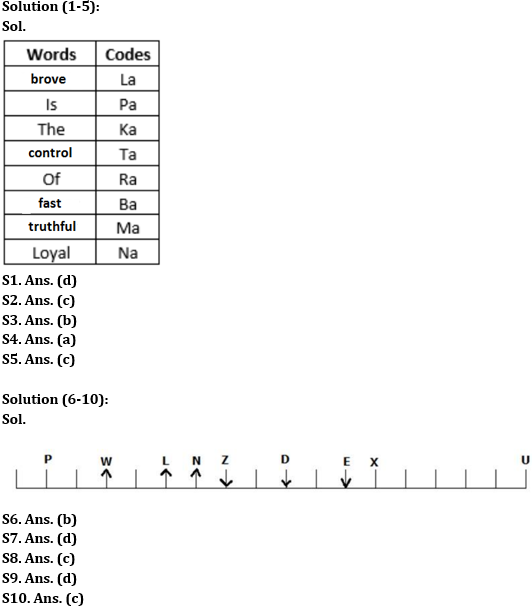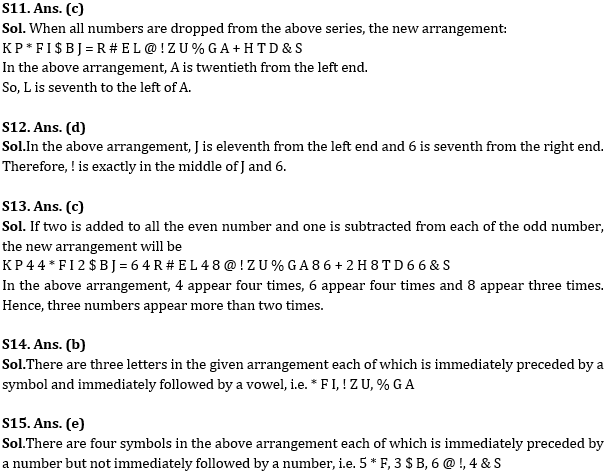Latest Banking jobs   »

# Reasoning Ability Quiz For RBI Grade B/ ECGC PO/ SIDBI Grade A Prelims 2022- 19th May

Directions (1-5): Study the following information carefully and answer the given questions.
In a certain code language,
‘Brove is the control of fast’ is coded as ‘la pa ka ta ra ba’
‘Truthful is the loyal’ is coded as ‘ma na pa ka’
‘Loyal the control of’ is coded as ‘na ka ra ta’
‘Truthful of fast’ is coded as ‘ma ra ba’

Q1. What is the code for ‘truthful’?
(a) ba
(b) pa
(c) na
(d) ma
(e) None of these

Q2. Which amongst the following word is coded as ‘ba’?
(a) brove
(b) Of
(c) fast
(d) truthful
(e) None of these

Q3. What are the codes for ‘brove control’?
(a) la ba
(b) la ta
(c) ra ta
(d) la ka
(e) None of these

Q4. What is the code for ‘Loyal’?
(a) na
(b) ka
(c) ta
(d) ra
(e) None of these

Q5. Which amongst the following words are coded as ‘ra ka’?
(a) Fast Of
(b) Of Loyal
(c) Of The
(d) The control
(e) None of these

Directions (6-10): Read the following information carefully to answer the questions based on it.
A certain number of persons sit in a linear row, some of them are facing in the north direction while others are facing in the south direction. The information for some of them is given. D faces in the south direction. W sits sixth to the right of D. P sits second to the left of W. L sits exactly between P and D. Z sits second to the right of L. E sits fourth to the left of Z. U sits sixth to the left of E and U sits at one of the ends in the row. Four persons sit between U and X, who sits sixth to the right of N. The number of persons sitting to the right of N is one less than twice of the persons sitting to the left of N.

Q6. How many persons are sitting in the row?
(a) 24
(b) 18
(c) 20
(d) 16
(e) None of these

Q7. Who sits just to the left of X?
(a) E
(b) P
(c) D
(e) None of these

Q8. How many persons sit between P and X?
(a) 8
(b) 9
(c) 10
(d) 11
(e) None of these

Q9. Which of the following statement is true?
(a) L sits fourth to the right of P.
(b) E sits sixth to the left of U.
(c) E sits just to the right of X.
(d) N sits exactly between L and Z.
(e) None of these

Q10. Who among the following sits exactly between Z and U?
(a) E
(b) D
(c) X
(e) None of these

Directions (11-15): Study the following alpha numeric symbol series and answers the questions given below:

K P 2 5 * F I 3 \$ B J = 4 5 R # E L 2 6 @ ! Z U % G A 9 4 + 3 H 6 T D 7 4 & S

Q11. If all numbers are dropped from the above series, then which of the following element will be 7th to the left of 20th element from the left end in the arrangement?
(a) #
(b) E
(c) L
(d) @
(e) None of these

Q12. Which of the following element is exactly in the middle of eleventh element from the left end and seventh from the right end?
(a) 2
(b) Z
(c) @
(d) !
(e) 6

Q13.If two is added to all the even number and one is subtracted from each of the odd number, then how many numbers in the new arrangement appears more than twice?
(a) One
(b) Two
(c) Three
(d) More than three
(e) None

Q14. How many such letters are there in the given arrangement each of which is immediately preceded by a symbol and immediately followed by a vowel?
(a) Four
(b) Three
(c) Two
(d) One
(e) None

Q15. How many such symbols are there in the given arrangement each of which is immediately preceded by a number but not immediately followed by a number?
(a)None
(b) One
(c) Two
(d) Three
(e) More than three

Solutions#### Congratulations!